# SCC Education

## Formulas for application of integral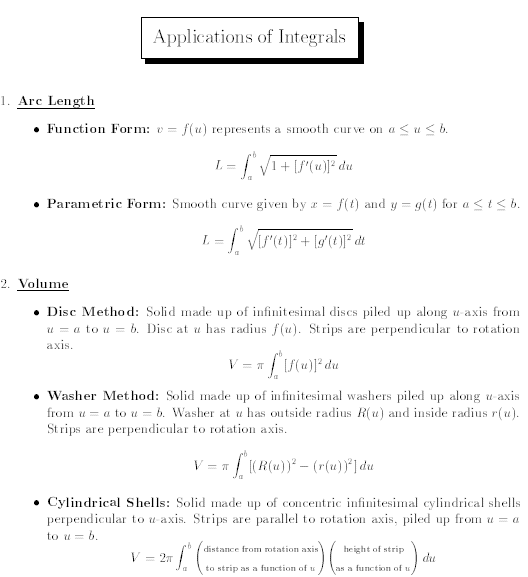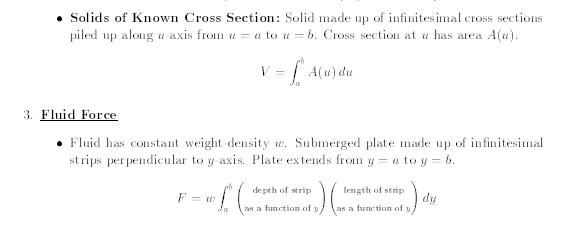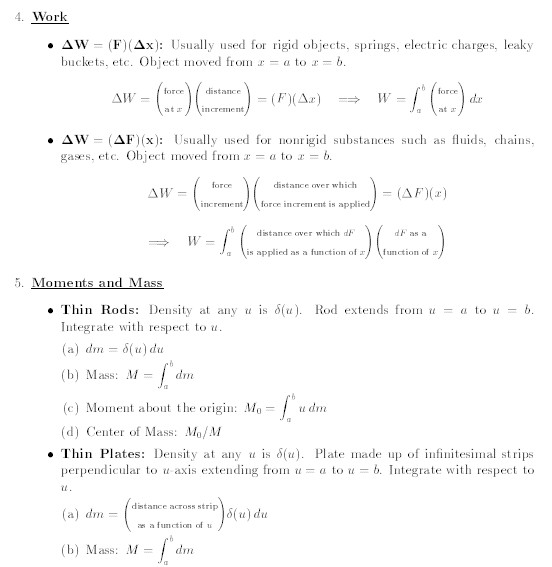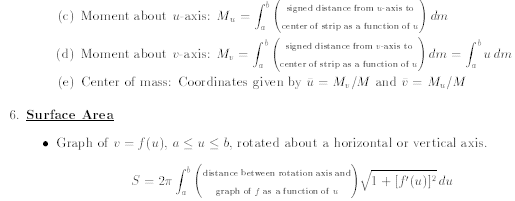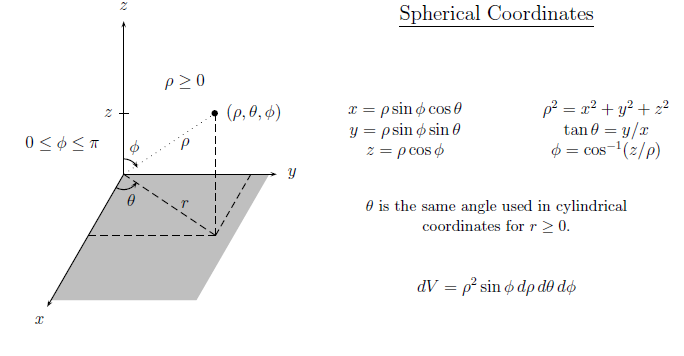introduction-of-hyperbolic-function

solving-models-related-to-ordinary differential equation

laplace-transform unit-step

the-inverse-hyperbolic-sine-inverse

multiple-integrals

l hopitals-rule

rules-of-differentiation partial

numerical-integration

critical-points-and-point-of-inflection

asymptotes

differentiation-of-vector

summary-of-convergence-test

runga-kutta-method

laplace-formulas

volume-of-solid-of-revolution

complex-integration

vector-calculus-questions

laplace-transforms-question

differential-equation-of-first-order

the-trapezium-rule

limit-continuity differentiability

numerical-differentiation

some-important-formulas-of-integration

solving-models-related-to-ordinary differential equation

differentiation-of-exponential-functions

area-between-curve

vector

application-of-derivatives

homogenous-differential-equation

inverse-trigonometry-questions

integration

integration-questions

Rolles-theorem-and-mean-value-theorem

Techniques-of-integration-substitution

critical-points-and-point-of-inflection

intermediate-value-theorem

### Sample paper Mathematics 12